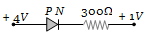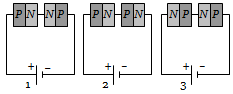In the forward bias arrangement of a PN-junction diode

(a) The N-end is connected to the positive terminal of the battery

(b) The P-end is connected to the positive terminal of the battery

(c) The direction of current is from N-end to P-end in the diode

(d) The P-end is connected to the negative terminal of battery

Concept Questions :-

PN junction
High Yielding Test Series + Question Bank - NEET 2020

Difficulty Level:

In a PN-junction diode

1. The current in the reverse biased condition is generally very small

2. The current in the reverse biased condition is small but the forward biased current is independent of the bias voltage

3. The reverse biased current is strongly dependent on the applied bias voltage

4. The forward biased current is very small in comparison to reverse biased current

Concept Questions :-

PN junction
High Yielding Test Series + Question Bank - NEET 2020

Difficulty Level:

The cut-in voltage for silicon diode is approximately

(a) 0.2 V                       (b) 0.6 V
(c) 1.1 V                       (d) 1.4 V

Concept Questions :-

Types of semiconductors
High Yielding Test Series + Question Bank - NEET 2020

Difficulty Level:

The electrical circuit used to get smooth output from a rectifier circuit is called

(a) Oscillator                          (b) Filter

(c) Amplifier                           (d) Logic gates

Concept Questions :-

Rectifier
High Yielding Test Series + Question Bank - NEET 2020

Difficulty Level:

PN-junction diode works as a insulator, if connected

(a) To A.C.                              (b) In forward bias
(c) In reverse bias                   (d) None of these

Concept Questions :-

Applications of PN junction
High Yielding Test Series + Question Bank - NEET 2020

Difficulty Level:

The reverse biasing in a PN junction diode

1. Decreases the potential barrier

2. Increases the potential barrier

3. Increases the number of minority charge carriers

4. Increases the number of majority charge carriers

Concept Questions :-

PN junction
High Yielding Test Series + Question Bank - NEET 2020

Difficulty Level:

The electrical resistance of depletion layer is large because

1. It has no charge carriers

2. It has a large number of charge carriers

3. It contains electrons as charge carriers

4. It has holes as charge carriers

Concept Questions :-

Types of semiconductors
High Yielding Test Series + Question Bank - NEET 2020

Difficulty Level:

In the circuit given below, the value of the current is(a) 0 amp                          (b) ${10}^{-2}$ amp
(c) ${10}^{2}$ amp                       (d) ${10}^{-3}$amp

Concept Questions :-

Applications of PN junction
High Yielding Test Series + Question Bank - NEET 2020

Difficulty Level:

The PN junction diode is used as

(a) An amplifier                       (b) A rectifier
(c) An oscillator                       (d) A modulator

Concept Questions :-

Applications of PN junction
High Yielding Test Series + Question Bank - NEET 2020

Difficulty Level:

Two PN-junctions can be connected in series by three different methods as shown in the figure. If the potential difference in the junctions is the same, then the correct connections will be(a) In the circuit (1) and (2)                    (b) In the circuit (2) and (3)
(c) In the circuit (1) and (3)                    (d) Only in the circuit (1)

Concept Questions :-

PN junction